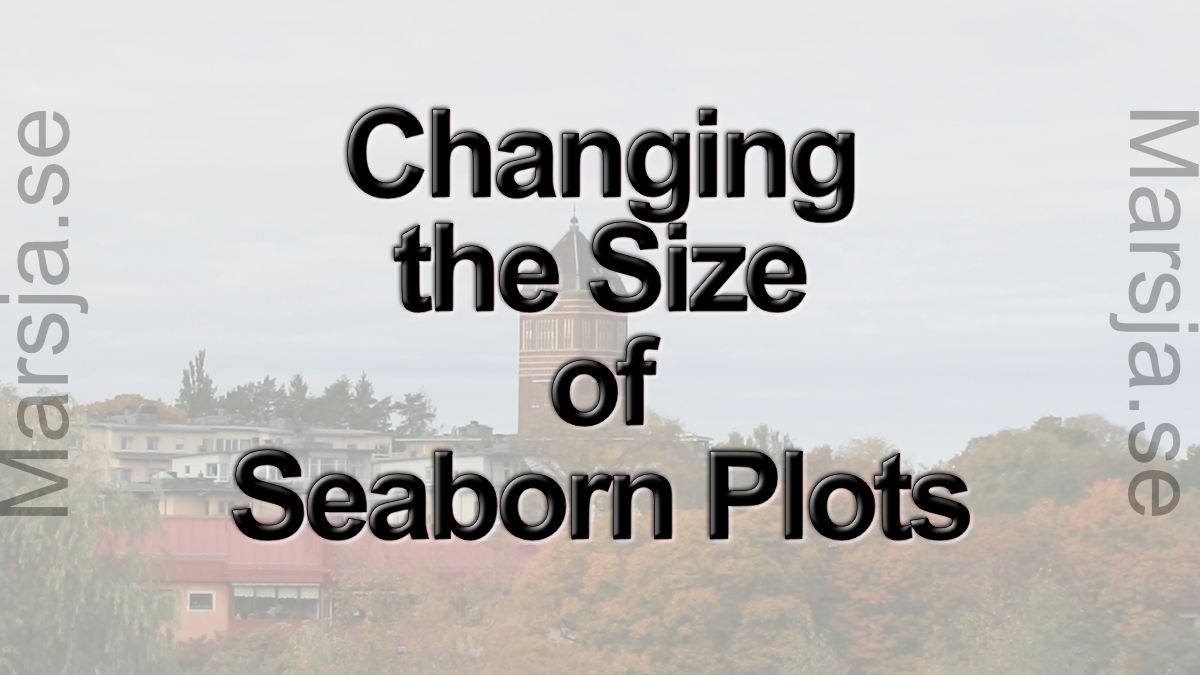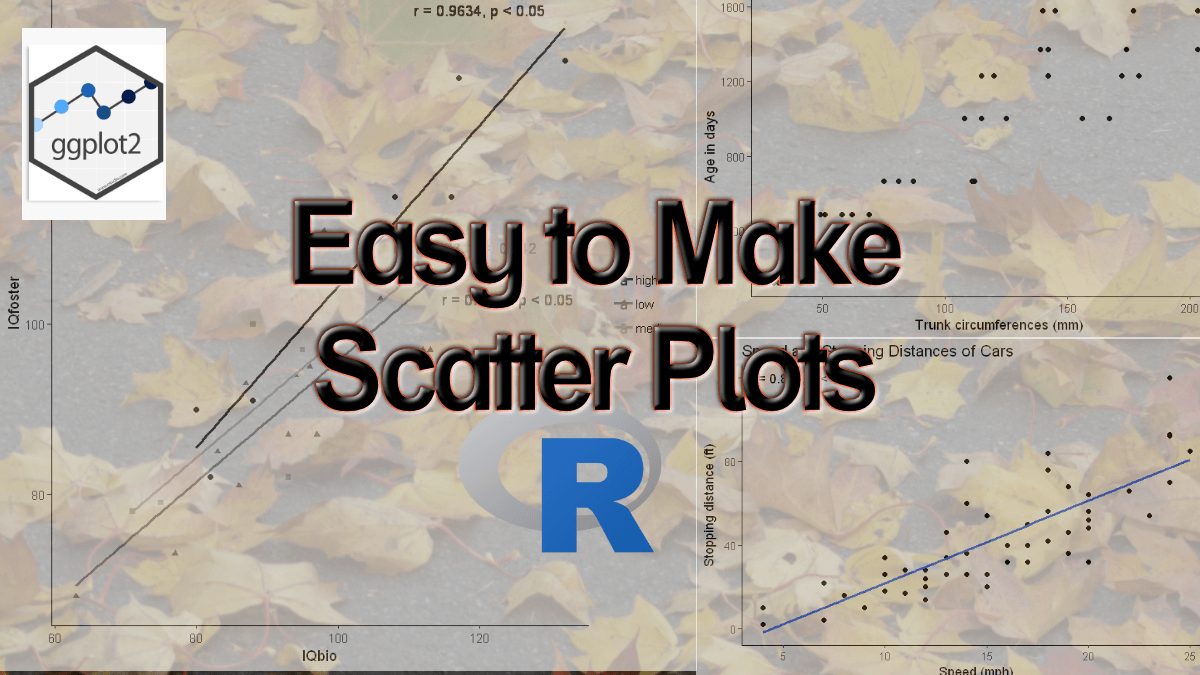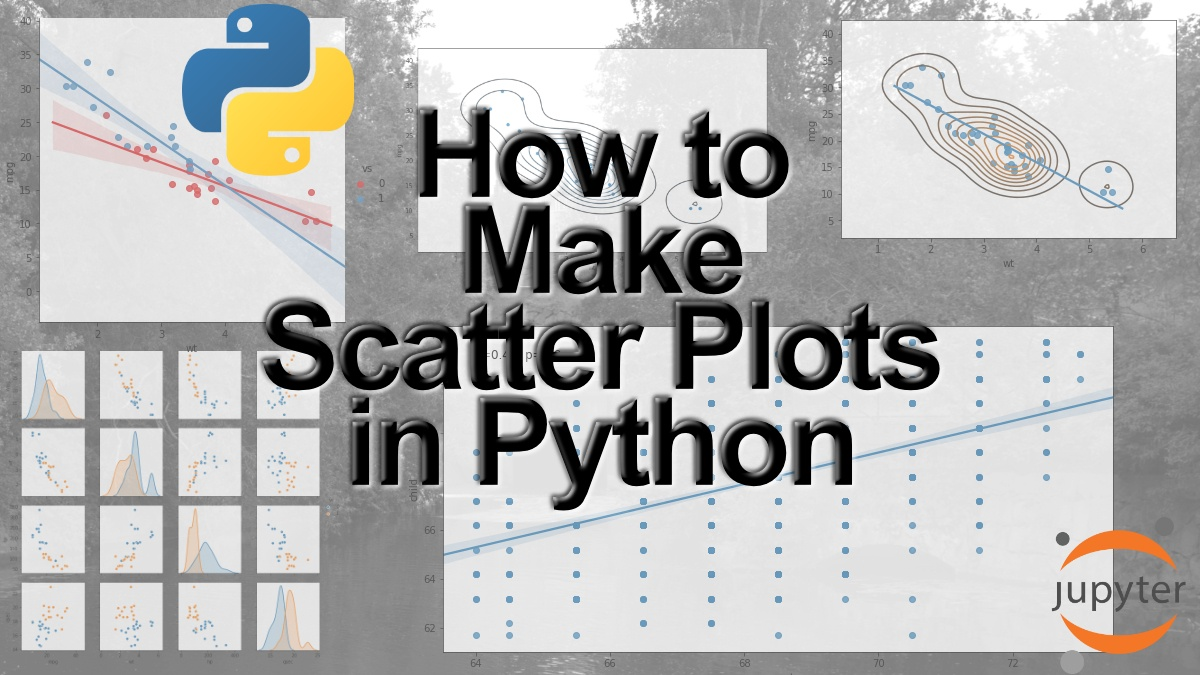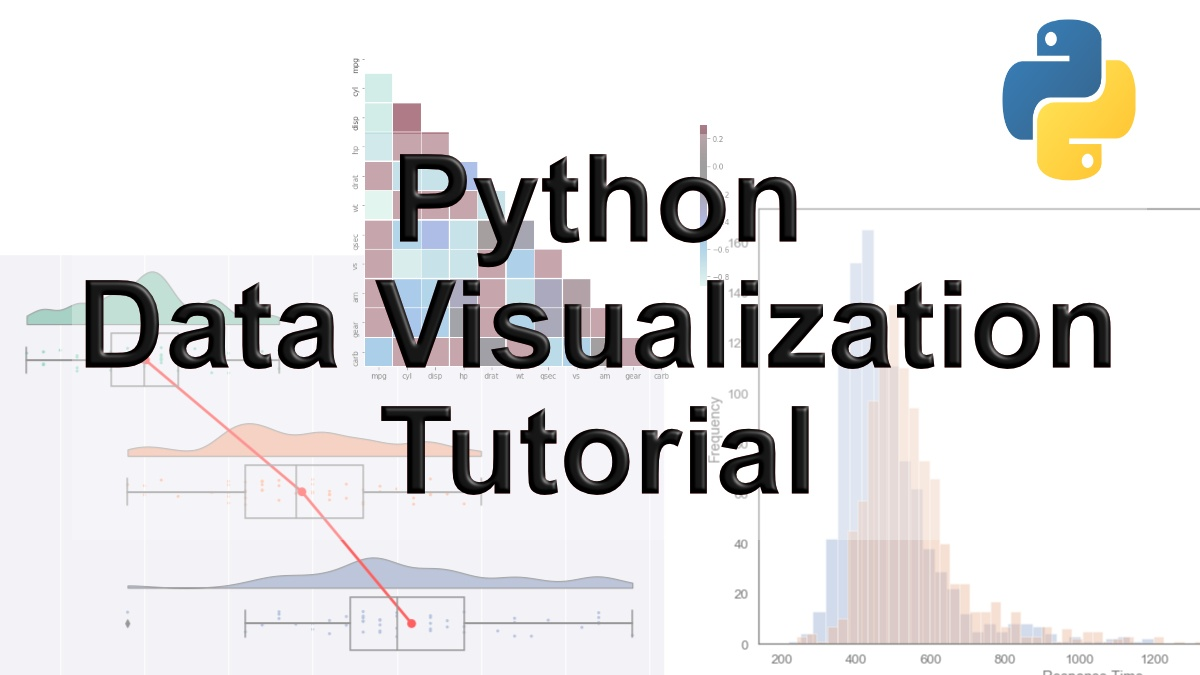## How to use Pandas Scatter Matrix (Pair Plot) to Visualize Trends in Data

In this Python data visualization tutorial, we will work with Pandas scatter_matrix method to explore trends in data. Previously, we have learned how to create scatter plots with Seaborn and histograms with Pandas, for instance. In this post, we’ll focus on scatter...## How to Change the Size of Seaborn Plots

In this short tutorial, we will learn how to change the figure size of Seaborn plots. For many reasons, we may need to either increase the size or decrease the size, of our plots created with Seaborn. How to Make Seaborn Plot Bigger Now, if we only want to know how to...## How to Make a Scatter Plot in R with Ggplot2

In this post, we will learn how make scatter plots using R and the package ggplot2. More specifically, we will learn how to make scatter plots, change the size of the dots, change the markers, the colors, and change the number of ticks.  Furthermore, we will...## How to Make a Scatter Plot in Python using Seaborn

Data visualization is a big part of the process of data analysis. In this post, we will learn how to make a scatter plot using Python and the package Seaborn. In detail, we will learn how to use the Seaborn methods scatterplot, regplot, lmplot, and pairplot to create...## 9 Data Visualization Techniques You Should Learn in Python

With the ever-increasing volume of data, it is impossible to tell stories without visualizations. Data visualization is an art of how to turn numbers into useful knowledge. Using Python we can learn how to create data visualizations and present data in Python using...